Next: 7.2.6 Performance outside the Up: 7.2 Methods Previous: 7.2.4 Forward model: Multi-layer

## 7.2.5 Forward model: Abstract recurrent neural network

The abstract RNN was used as an alternative to the MLP. Tests were carried out on two data sets, called standard' and change'. The first is the same as for the MLP. In the second set, the predicted sensory state St+1 is replaced by the relative change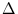St = St+1 - St. Thus, the network output needs to be added to the current sensory state to obtain the predicted state.

For the training, MPPCA-ext, NGPCA, and NGPCA-constV were used (chapter 3). The number of units was 50. NGPCA and NGPCA-constV had the same training parameters as in chapter 4, namely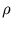(0) = 10.0,(tmax) = 0.0001,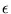(0) = 0.5,(tmax) = 0.001, and tmax = 400 000.

To get an estimate of the number q of principal components needed for each training set, the local dimensionality of the pattern distribution was computed. This was done as in section 4.5. The peak in the ratio of successive averaged eigenvalues--from a local PCA within the neighborhood of each training pattern--was determined. Here, the number of neighbors differed from the one given in section 4.5. This number needs to be sufficiently large to point out the underlying dimensionality (figure 7.7, left). For the standard set, the peak in the eigenvalue ratio is at four (figure 7.7, left). As expected, the robot's location (distance from the circle center), its orientation, and the two velocities make four degrees of freedom. For the change set, however, this peak was at 13 (figure 7.7, right). Here, the explanation is that the noise-to-signal ratio is higher; the noise inSt equals about the noise in St, but the magnitude ofSt is about ten-times smaller than the magnitude of St. The additional variance increases the local dimensionality.

Figure 7.7: Ratio of successive averaged eigenvalues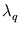and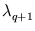. For different numbers k of neighbors, results are shown for the standard training set (left) and the change set (right).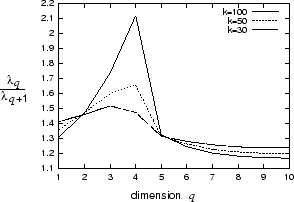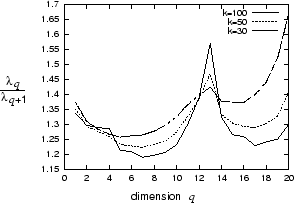The mixture models were tested with q = 5 for the standard set and with q = 14 for the change set. As in section 6.2.5, one principal component was added to take care of the curvature of the distribution. This improved the performance.

As shown in chapter 4, the abstract RNN can associate patterns in any direction; the MLP is restricted to the trained direction. To demonstrate this advantage of the RNN, another experiment used the same trained RNN as an inverse model. Here, two successive states St and St+1 are mapped onto the motor command Mt. The performance of the abstract RNN is shown in section 7.3.2.Next: 7.2.6 Performance outside the Up: 7.2 Methods Previous: 7.2.4 Forward model: Multi-layer
Heiko Hoffmann
2005-03-22